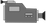# TI-84: Generating Random Numbers

UpdatedTI-84 Video: Generating Random Numbers  (YouTube) (Vimeo)

## 1. Be sure you "seed" your calculator. This will ensure that the same random numbers do not appear on everyone's calculator. Enter a random number in the calculator such as the student ID number or telephone number. Then press [STO->] [MATH] "PRB" "1:rand".

Note: This step only needs to be completed once unless you reset the calculator! Â And you will not get the same numbers!## 2. Specify the minimum and maximum integers possible, and how many random integers to generate. For example, [MATH] "PRB" "5:randInt(1,10,5)" [ENTER] generates 5 numbers between 1 and 10.

Note: Hit [Enter] to get 5 more random numbers between 1 and 10. Of course, you will not likely get the same random numbers.## 3. To simulate flipping a coin, let '0' be heads and '1' be tails. For example, [MATH] "PRB" "5:randInt(0,1,6)" [ENTER] generates 6 numbers between 0 and 1. All of the '0's are Heads. All of the '1's are Tails!

The image below shows 3 heads and 3 tails. Then it shows 4 heads and 2 tails.## 4. To simulate rolling dice, go to [MATH] "PRB" "5:randInt(1,6,2)" [ENTER] generates 2 numbers between 1 and 6.

The image below shows rolling a die three times and the results.## 5. You could also generate a lot of numbers and store them in a list. For example, [MATH] "PRB" "5:randInt(1,10,100" [ENTER] generates 100 numbers between 1 and 10. Then [STO->] [2nd] 'L1' will place the numbers in List 1.